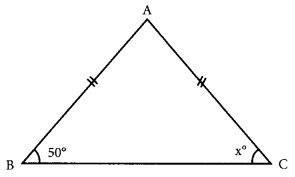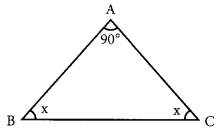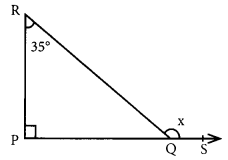# MCQ Questions for Class 7 Maths Chapter 6 The Triangles and its Properties with Answers

We have compiled the NCERT MCQ Questions for Class 7 Maths Chapter 6 The Triangles and its Properties with Answers Pdf free download covering the entire syllabus. Practice MCQ Questions for Class 7 Maths with Answers on a daily basis and score well in exams. Refer to the The Triangles and its Properties Class 7 MCQs Questions with Answers here along with a detailed explanation.

## The Triangles and its Properties Class 7 MCQs Questions with Answers

Choose the correct option.

Question 1.
How many elements are there in a triangle?
(a) 3
(b) 4
(c) 6
(d) 8

Question 2.
If two sides of a triangle are not equal the triangle is called
(a) scalene
(b) isosceles
(c) equilateral
(d) right-angled

Question 3.
If all the three sides of a triangle are equal, the triangle is called
(a) equilateral
(b) right-angled
(c) isosceles
(d) scalene

Question 4.
If one angle of a triangle is obtuse, the triangle is called
(a) acute-angled
(b) right-angled
(c) scalene
(d) obtuse-angled

Question 5.
How many medians can a triangle have?
(a) 1
(b) 2
(c) 3
(d) 6

Question 6.
In a right triangle, if hypotenuse is H, perpendicular is P and base is B then
(a) B² = H² + P²
(b) H² = P² + B²
(c) H² = P² – B²
(d) P² = B² + H²

Answer: (b) H² = P² + B²

Question 7.
The measure of each angle of an equilateral triangle is
(a) 30°
(b) 60°
(c) 90°
(d) 45°

Question 8.
Which of the following cannot be the sides of a right triangle?
(a) 2 cm, 2 cm, 4 cm
(b) 5 cm, 12 cm; 13 cm
(c) 6 cm, 8 cm, 10 cm
(d) 3 cm; 4 cm; 5 cm

Answer: (a) 2 cm, 2 cm, 4 cm

Question 9.
Find the angle x in the given figure.(a) 40°
(b) 45°
(c) 50°
(d) 60°

Question 10.
In the given figure, find ‘x’(a) 60°
(b) 70°
(c) 80°
(d) 75°

Question 11.
Least number of possible acute angles in a triangle is:
(a) 0
(b) 1
(c) 2
(d) 3

Question 12.
Maximum number of possible obtuse angles in a triangle is:
(a) 0
(b) 1
(c) 2
(d) 3

Fill in the blanks

Question 1.
There can be only ……………… obtuse angles in a triangle.

Question 2.In the given ∆ABC, ∠x = ………………

Question 3.In the given ∆PQR, ∠x is ……………….

Question 4.
In a triangle, the sum of the lengths of any two sides can never be …………….. than the length of third side.

Question 5.
If two sides of a triangle are equal then it is called an ……………….

Question 6.
For a ΔABC, ∠A + ∠B + ∠C = …………………..

Question 7.
The measure of each angle of an equilateral triangle is …………………

Question 8.
In a right triangle, the side opposite to 90° is called ………………. [hypotenuse/base]

Question 9.
The perpendicular line segment drawn from a vertex of a triangle to the opposite side is called its ………………….. [median/altitude]

Question 10.
If the measure of each side of a triangle is the same, then the triangle is called an …………………. triangle. [equilateral/isosceles]

Question 11.
If the two sides of a triangle are equal then it is called an …………………….. triangle. [equilateral/isosceles]

Question 12.
There can be only …………………… acute angle(s) of a triangle. [three/two]

Question 13.
There can be only …………………… obtuse angle(s) of a triangle. [one/two]

Question 14.
40°, 50° and 80° ………………….. be the angles of a triangle. [can/cannot]

Question 15.
30°, 90° and 90° ……………….. be the angles of a triangle. [can/cannot]

Question 16.
5 cm, 3.8 cm and 9.1 cm …………………… be the measures of the sides of a triangle. [can/cannot]